# Poisson Noise Reduction with Non-local PCA

## Abstract

Photon-limited imaging arises when the number of photons collected by a sensor array is small relative to the number of detector elements. Photon limitations are an important concern for many applications such as spectral imaging, night vision, nuclear medicine, and astronomy. Typically a Poisson distribution is used to model these observations, and the inherent heteroscedasticity of the data combined with standard noise removal methods yields significant artifacts. This paper introduces a novel denoising algorithm for photon-limited images which combines elements of dictionary learning and sparse patch-based representations of images. The method employs both an adaptation of Principal Component Analysis (PCA) for Poisson noise and recently developed sparsity-regularized convex optimization algorithms for photon-limited images. A comprehensive empirical evaluation of the proposed method helps characterize the performance of this approach relative to other state-of-the-art denoising methods. The results reveal that, despite its conceptual simplicity, Poisson PCA-based denoising appears to be highly competitive in very low light regimes.

This is a preview of subscription content, log in to check access.

## Access options

US$39.95 Price includes VAT for USA ### Subscribe to journal Immediate online access to all issues from 2019. Subscription will auto renew annually. US$ 99

This is the net price. Taxes to be calculated in checkout.

## References

1. 1.

Aharon, M., Elad, M., Bruckstein, A.: K-SVD: an algorithm for designing overcomplete dictionaries for sparse representation. IEEE Trans. Signal Process. 54(11), 4311–4322 (2006)

2. 2.

Anscombe, F.J.: The transformation of Poisson, binomial and negative-binomial data. Biometrika 35, 246–254 (1948)

3. 3.

Banerjee, A., Merugu, S., Dhillon, I.S., Ghosh, J.: Clustering with Bregman divergences. J. Mach. Learn. Res. 6, 1705–1749 (2005)

4. 4.

Borkowski, K.J., Reynolds, S.P., Green, D.A., Hwang, U., Petre, R., Krishnamurthy, K., Willett, R.: Radioactive Scandium in the youngest galactic supernova remnant G1.9+0.3. Astrophys. J. Lett. 724, L161 (2010)

5. 5.

Boulanger, J., Kervrann, C., Bouthemy, P., Elbau, P., Sibarita, J.-B., Salamero, J.: Patch-based nonlocal functional for denoising fluorescence microscopy image sequences. IEEE Trans. Med. Imaging 29(2), 442–454 (2010)

6. 6.

Boyd, S., Parikh, N., Chu, E., Peleato, B., Eckstein, J.: Distributed optimization and statistical learning via the alternating direction method of multipliers. Found. Trends Mach. Learn. 3(1), 1–122 (2011)

7. 7.

Bregman, L.M.: The relaxation method of finding the common point of convex sets and its application to the solution of problems in convex programming. Comput. Math. Math. Phys. 7(3), 200–217 (1967)

8. 8.

Buades, A., Coll, B., Morel, J.-M.: A review of image denoising algorithms, with a new one. Multiscale Model. Simul. 4(2), 490–530 (2005)

9. 9.

Chatterjee, P., Milanfar, P.: Patch-based near-optimal image denoising. In: ICIP (2011)

10. 10.

Collins, M., Dasgupta, S., Schapire, R.E.: A generalization of principal components analysis to the exponential family. In: NIPS, pp. 617–624 (2002)

11. 11.

Dabov, K., Foi, A., Katkovnik, V., Egiazarian, K.O.: Image denoising by sparse 3-D transform-domain collaborative filtering. IEEE Trans. Image Process. 16(8), 2080–2095 (2007)

12. 12.

Dabov, K., Foi, A., Katkovnik, V., Egiazarian, K.O.: BM3D image denoising with shape-adaptive principal component analysis. In: Proc. Workshop on Signal Processing with Adaptive Sparse Structured Representations (SPARS’09) (2009)

13. 13.

Danielyan, A., Foi, A., Katkovnik, V., Egiazarian, K.: Denoising of multispectral images via nonlocal groupwise spectrum-PCA. In: CGIV2010/MCS’10, pp. 261–266 (2010)

14. 14.

Deledalle, C.-A., Denis, L., Tupin, F.: Poisson NL means: Unsupervised non local means for Poisson noise. In: ICIP, pp. 801–804 (2010)

15. 15.

Deledalle, C.-A., Salmon, J., Dalalyan, A.S.: Image denoising with patch based PCA: local versus global. In: BMVC (2011)

16. 16.

Figueiredo, M.A.T., Bioucas-Dias, J.M.: Restoration of poissonian images using alternating direction optimization. IEEE Trans. Signal Process. 19(12), 3133–3145 (2010)

17. 17.

Fisz, M.: The limiting distribution of a function of two independent random variables and its statistical application. Colloq. Math. 3, 138–146 (1955)

18. 18.

Fryźlewicz, P., Nason, G.P.: Poisson intensity estimation using wavelets and the Fisz transformation. Technical report, Department of Mathematics, University of Bristol, United Kingdom (2001)

19. 19.

Gordon, G.J.: Generalized2 linear2 models. In: NIPS, pp. 593–600 (2003)

20. 20.

Harmany, Z., Marcia, R., Willett, R.: This is SPIRAL-TAP: sparse poisson intensity reconstruction algorithms—theory and practice. IEEE Trans. Image Process. 21(3), 1084–1096 (2012)

21. 21.

Katkovnik, V., Foi, A., Egiazarian, K.O., Astola, J.T.: From local kernel to nonlocal multiple-model image denoising. Int. J. Comput. Vis. 86(1), 1–32 (2010)

22. 22.

Kervrann, C., Boulanger, J.: Optimal spatial adaptation for patch-based image denoising. IEEE Trans. Image Process. 15(10), 2866–2878 (2006)

23. 23.

Kolaczyk, E.D.: Wavelet shrinkage estimation of certain Poisson intensity signals using corrected thresholds. Stat. Sin. 9(1), 119–135 (1999)

24. 24.

Kolaczyk, E.D., Nowak, R.D.: Multiscale likelihood analysis and complexity penalized estimation. Ann. Stat. 32(2), 500–527 (2004)

25. 25.

Krishnamurthy, K., Raginsky, M., Willett, R.: Multiscale photon-limited spectral image reconstruction. SIAM J. Imaging Sci. 3(3), 619–645 (2010)

26. 26.

Lebrun, M., Colom, M., Buades, A., Morel, J.-M.: Secrets of image denoising cuisine. Acta Numer. 21(1), 475–576 (2012)

27. 27.

Lee, H., Battle, A., Raina, R., Ng, A.Y.: Efficient sparse coding algorithms. In: NIPS, pp. 801–808 (2007)

28. 28.

Maggioni, M., Katkovnik, V., Egiazarian, K., Foi, A.: A nonlocal transform-domain filter for volumetric data denoising and reconstruction. IEEE Trans. Image Process. 22, 119–133 (2013)

29. 29.

Mairal, J., Bach, F., Ponce, J., Sapiro, G.: Online learning for matrix factorization and sparse coding. J. Mach. Learn. Res., 19–60 (2010)

30. 30.

Mairal, J., Bach, F., Ponce, J., Sapiro, G., Zisserman, A.: Non-local sparse models for image restoration. In: ICCV, pp. 2272–2279 (2009)

31. 31.

Mäkitalo, M., Foi, A.: Optimal inversion of the Anscombe transformation in low-count Poisson image denoising. IEEE Trans. Image Process. 20(1), 99–109 (2011)

32. 32.

Mäkitalo, M., Foi, A.: Optimal inversion of the generalized Anscombe transformation for Poisson-Gaussian noise. IEEE Trans. Image Process. 22, 91–103 (2013)

33. 33.

Muresan, D.D., Parks, T.W.: Adaptive principal components and image denoising. In: ICIP, pp. 101–104 (2003)

34. 34.

Nielsen, F., Garcia, V.: Statistical exponential families: a digest with flash cards. Arxiv preprint (2009). arXiv:0911.4863

35. 35.

Roy, N., Gordon, G.J., Thrun, S.: Finding approximate POMDP solutions through belief compression. J. Artif. Intell. Res. 23(1), 1–40 (2005)

36. 36.

Salmon, J., Deledalle, C.-A., Willett, R., Harmany, Z.: Poisson noise reduction with non-local PCA. In: ICASSP (2012)

37. 37.

Salmon, J., Strozecki, Y.: Patch reprojections for non local methods. Signal Process. 92(2), 447–489 (2012)

38. 38.

Salmon, J., Willett, R., Arias-Castro, E.: A two-stage denoising filter: the preprocessed Yaroslavsky filter. In: SSP (2012)

39. 39.

Singh, A.P., Gordon, G.J.: Relational learning via collective matrix factorization. In: Proceeding of the 14th ACM SIGKDD International Conference on Knowledge Discovery and Data Mining, pp. 650–658. ACM, New York (2008)

40. 40.

Singh, A.P., Gordon, G.J.: A unified view of matrix factorization models. In: Machine Learning and Knowledge Discovery in Databases, pp. 358–373. Springer, Berlin (2008)

41. 41.

Willett, R.: Multiscale analysis of photon-limited astronomical images. In: Statistical Challenges in Modern Astronomy (SCMA) IV (2006)

42. 42.

Willett, R., Nowak, R.D.: Platelets: a multiscale approach for recovering edges and surfaces in photon-limited medical imaging. IEEE Trans. Med. Imaging 22(3), 332–350 (2003)

43. 43.

Willett, R., Nowak, R.D.: Fast multiresolution photon-limited image reconstruction. In: Proc. IEEE Int. Sym. Biomedical Imaging—ISBI ’04 (2004)

44. 44.

Wright, S.J., Nowak, R.D., Figueiredo, M.A.T.: Sparse reconstruction by separable approximation. IEEE Trans. Signal Process. 57(7), 2479–2493 (2009)

45. 45.

Yin, W., Osher, S., Goldfarb, D., Darbon, J.: Bregman iterative algorithms for l1-minimization with applications to compressed sensing. SIAM J. Imaging Sci. 1(1), 143–168 (2008)

46. 46.

Zhang, B., Fadili, J., Starck, J.-L.: Wavelets, ridgelets, and curvelets for Poisson noise removal. IEEE Trans. Image Process. 17(7), 1093–1108 (2008)

47. 47.

Zhang, L., Dong, W., Zhang, D., Shi, G.: Two-stage image denoising by principal component analysis with local pixel grouping. Pattern Recognit. 43(4), 1531–1549 (2010)

48. 48.

Zou, H., Hastie, T., Tibshirani, R.: Sparse principal component analysis. J. Comput. Graph. Stat. 15(2), 265–286 (2006)

## Acknowledgements

Joseph Salmon, Zachary Harmany, and Rebecca Willett gratefully acknowledge support from DARPA grant no. FA8650-11-1-7150, AFOSR award no. FA9550-10-1-0390, and NSF award no. CCF-06-43947. The authors would also like to thank J. Boulanger and C. Kervrann for providing their SAFIR algorithm, Steven Reynolds for providing the spectral images from the supernova remnant G1.9+0.3, and an anonymous reviewer for proposing the improvement using the binning step.

## Author information

Correspondence to Joseph Salmon.

## Appendices

### Lemma 1

The function L is biconvex with respect to (U,V) but not jointly convex.

### Proof

The biconvexity argument is straightforward; the partial functions UL(U,V) with a fixed V and VL(U,V) with a fixed U are both convex. The fact that the problem is non-jointly convex can be seen when U and V are in $$\mathbb{R}$$ (i.e.=m=n=1), since the Hessian in this case is

$$H_L(U,V) = \begin{pmatrix} V^2 e^{UV} \ \ & UV e^{UV}+e^{UV}-Y\\ UV e^{UV}+e^{UV}-Y \ \ & U^2 e^{UV} \end{pmatrix} .$$

Thus at the origin one has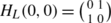, which has a negative eigenvalue, −1. □

We provide below the gradient computation used in Eqs. (13) and (3):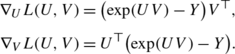Using the component-wise representation this is equivalent to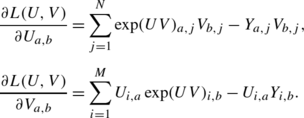### Appendix C: Hessian Calculations

The approach proposed by [19, 35] consists in using an iterative algorithm which sequentially updates the jth column of V and the ith row of U. The only problem with this method is numerical: one needs to invert possibly ill conditioned matrices at each step of the loop.

The Hessian matrices of our problems, with respect to U and V respectively are given by

$$\frac{\partial^2 L(U,V)}{\partial U_{a,b} \partial U_{c,d}}=\left \{ \begin{array}{l@{\ \ }l} \sum_{j=1}^N \exp(UV)_{a,j} V^2_{b,j}, & \mbox{if } (a,b)=(c,d), \\[3pt] 0 & \mbox{otherwise,} \end{array} \right .$$

and

$$\frac{\partial^2 L(U,V)}{\partial V_{a,b} \partial V_{c,d}}=\left \{ \begin{array}{l@{\ \ }l} \sum_{i=1}^M U^2_{i,a} \exp(UV)_{i,b}, &\mbox{if } (a,b)=(c,d), \\[3pt] 0&\mbox{otherwise.} \end{array} \right .$$

Notice that both Hessian matrices are diagonal. So applying the inverse of the Hessian simply consists in inverting the diagonal coefficients.

### Appendix D: The Newton Step

In the following we need to introduce the function $$\operatorname{Vect}_{C}$$ that transforms a matrix into one single column (concatenates the columns), and the function $$\operatorname{Vect}_{R}$$ that transforms a matrix into a single row (concatenates the rows). This means that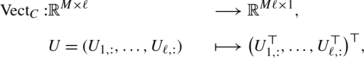and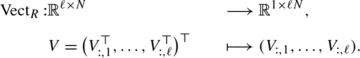Now using the previously introduced notations, the updating steps for U and V can be written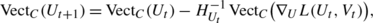(25)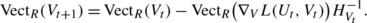(26)

We give the order used to concatenate the coefficients for the Hessian matrix with respect to U, H U : (a,b)=(1,1),…,(M,1),(1,2),…(M,2),…(1,),…,(M,). We concatenate the column of U in this order.

It is easy to give the updating rules for the kth column of U, one only needs to multiply the first equation of (25) from the left by the M×Mℓ matrix

$$F_{k,M,\ell,} = \begin{pmatrix} 0_{M,M}, & \ldots,& I_{M,M},&\ldots,& 0_{M,M} \end{pmatrix}$$
(27)

where the identity block matrix is in the kth position. This leads to the following updating rule

$${U_{t+1,\cdot,k}=U_{t,:,k} -D_k^{-1} \bigl( \exp(U_tV_t) -Y\bigr)V_{t,k,:}^\top } ~,$$
(28)

where D k is a diagonal matrix of size M×M: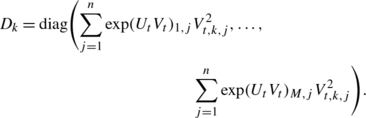By the symmetry of the problem in U and V, one has the following equivalent updating rule for V:

$${V_{t+1,k,:}=V_{t,k,:} -U_{t,:,k}^\top\bigl( \exp(U_tV_t) -Y\bigr) E_{k,M}^{-1} } ~,$$
(29)

where E k is a diagonal matrix of size N×N: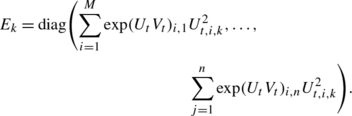## Rights and permissions

Reprints and Permissions

Salmon, J., Harmany, Z., Deledalle, C. et al. Poisson Noise Reduction with Non-local PCA. J Math Imaging Vis 48, 279–294 (2014) doi:10.1007/s10851-013-0435-6